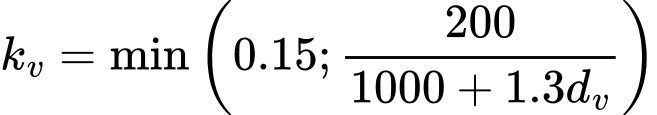The link was sent to your email.

Tree
Settings
Product:
Program:
Language:

## Verification of rectangular RC cross-section

The cross-section is rectangular, unilaterally reinforced and loaded by the bending moment and normal compression force. The program verifies a reinforced concrete section using the method of limit deformation. The maximum allowable strain of concrete in compression is 0,002 - 0,0035. Compression reinforcement is not taken into account.

The computed degree of reinforcement is checked using the following expressions:where: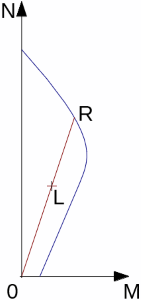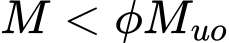Interaction diagram N-M

Usage ratio of concrete cross-section subject to the combination of bending moment and normal force is determined as |0L| / |0R|. Where L is load and R is strength with prescribed excentricity.

## Bending without normal force

The cross-section is rectangular, reinforced on one side and loaded by the bending moment M.

The permissible moment for a given area of reinforcements As reads: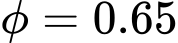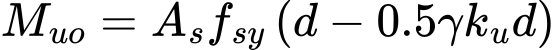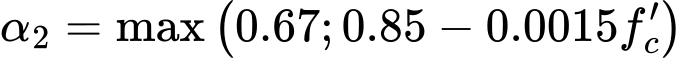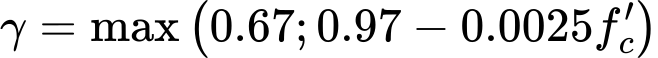The program further checks whether the neutral axis parameter ku is less than the limit value: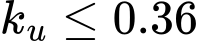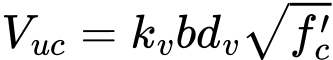where: x - depth of neutral axis

## Shear

The program further checks ultimate shear strength: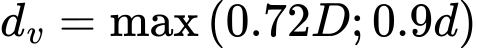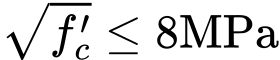where: